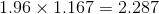# AP Statistics : How to find confidence intervals for a mean

## Example Questions

### Example Question #1 : Confidence Intervals

Suppose you have a normally distributed variable with known variance. How many standard errors do you need to add and subtract from the sample mean so that you obtain 95% confidence intervals?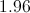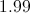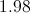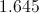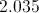Explanation:

To obtain 95% confidence intervals for a normal distribution with known variance, you take the mean and add/subtract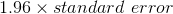. This is because 95% of the values drawn from a normally distributed sampling distribution lie within 1.96 standard errors from the sample mean.

### Example Question #1 : Confidence Intervals

An automotive engineer wants to estimate the cost of repairing a car that experiences a 25 MPH head-on collision. He crashes 24 cars, and the average repair is $11,000. The standard deviation of the 24-car sample is$2,500.

Provide a 98% confidence interval for the true mean cost of repair.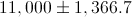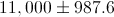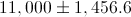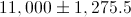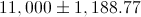Explanation:

Standard deviation for the samle mean: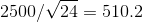Since n < 30, we must use the t-table (not the z-table).

The 98% t-value for n=24 is 2.5.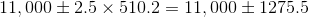### Example Question #3 : Confidence Intervals

300 hundred eggs were randomly chosen from a gravid female salmon and individually weighed. The mean weight was 0.978 g with a standard deviation of 0.042. Find the 95% confidence interval for the mean weight of the salmon eggs (because it is a large n, use the standard normal distribution).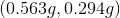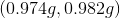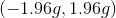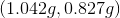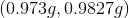Explanation:

Because we have such a large sample size, we are using the standard normal or z-distribution to calculate the confidence interval.

Formula: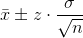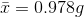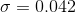We must find the appropriate z-value based on the given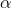for 95% confidence: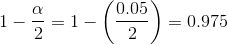Then, find the associated z-score using the z-table for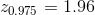Now we fill in the formula with our values from the problem to find the 95% CI.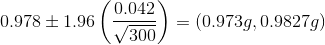### Example Question #4 : Confidence Intervals

A sample of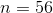observations of 02 consumption by adult western fence lizards gave the following statistics: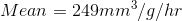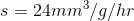Find the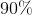confidence limit for the mean 02 consumption by adult western fence lizards.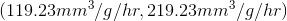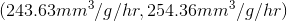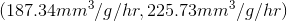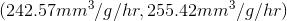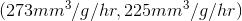Explanation:

Because we are only given the sample standard deviation we will use the t-distribution to calculate the confidence interval.

Appropriate Formula: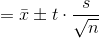Now we must identify our variables: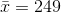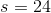We must find the appropriate t-value based on the givent-value at 90% confidence: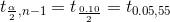Look up t-value for 0.05, 55 , so t-value= ~ 1.6735

90% CI becomes: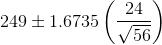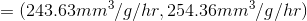### Example Question #5 : Confidence Intervals

 Subject Horn Length (in) Subject Horn Length (in) 1 19.1 11 11.6 2 14.7 12 18.5 3 10.2 13 28.7 4 16.1 14 15.3 5 13.9 15 13.5 6 12.0 16 7.7 7 20.7 17 17.2 8 8.6 18 19.0 9 24.2 19 20.9 10 17.3 20 21.3

The data above represents measurements of the horn lengths of African water buffalo that were raised on calcium supplements. Construct a 95% confidence interval for the population mean for horn length after supplments.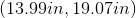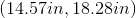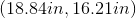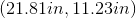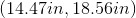Explanation:

First you must calculate the sample mean and sample standard deviation of the sample.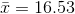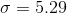Because we do not know the population standard deviation we will use the t-distribution to calculate the confidence intervals. We must use standard error in this formula because we are working with the standard deviation of the sampling distribution.

Formula: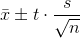To find the appropriate t-value for 95% confidence interval: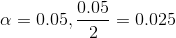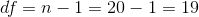Look up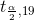in t-table and the corresponding t-value = 2.093.

Thus the 95% confidence interval is: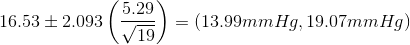### Example Question #1 : Confidence Intervals

The population standard deviation is 7. Our sample size is 36.

What is the 95% margin of error for:

1) the population mean

2) the sample mean

1) 14.567

2) 4.445

1) 12.266

2) 3.711

1) 15.554

2) 3.656

1) 11

2) 3

1) 13.720

2) 2.287

1) 13.720

2) 2.287

Explanation:

For 95% confidence, Z = 1.96.

1) The population M.O.E. =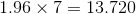2) The sample standard deviation =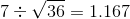The sample M.O.E. =